# Know the GMAT Code: Mixed-Shape Geometry

by , Nov 18, 2016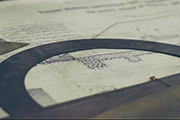Geometry can be fairly straightforward on the GMAT. It can also be seriously convoluted. Were going to talk about one of the big ways that the GMAT test writers make geometry really hard: by mixing different shapes together.

Try out this problem in our Know the Code series and then well dig in to figure out whats going on. Note: this ones from the GMATPrep free exams.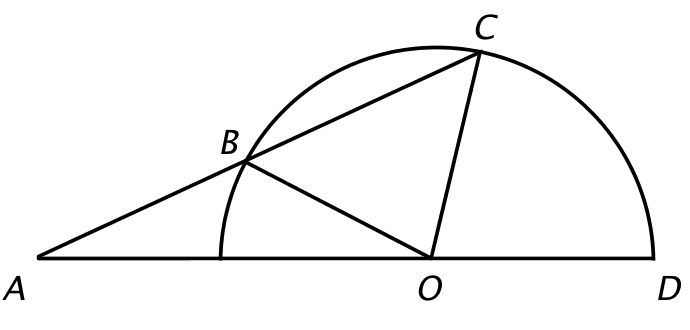*In the figure shown, point O is the center of the semicircle and points B, C, and D lie on the semicircle. If the length of line segment AB is equal to the length of line segment OC, what is the degree measure of angle BAO?

(1) The degree measure of angle COD is 60.

(2) The degree measure of angle BCO is 40.

(If you are new to Data Sufficiency and wondering where the answer choices are, start here and come back to this article later.)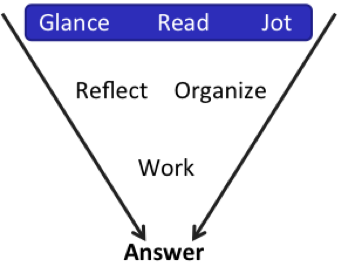1-second Glance. Geometry. DS. Ugly shape. Lots of text.

Read. Wow. Okay, Im going to re-draw this first so that I can add (jot) stuff from the text as I go. Lets see. Lines OC and OB are radii of the circle, so theyre the same length. Oh, and they tell me that AB is the same length as OC, so AB is like another radius.

[Aside: why am I making that connection to the radius vs. just thinking that theyre the same length? Because I know, from experience, that mixed shape problems often have some hidden connections; the key to the problem is usually about finding those connections.]

They want to know angle BAO.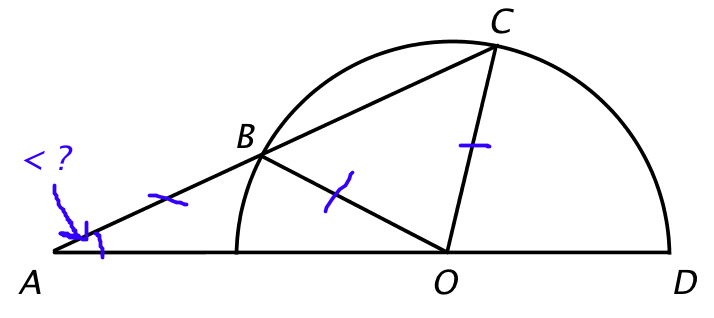Can we infer anything from that information?

Yes! Within any one triangle, if two sides are the same, then the angles opposite those sides are also the same. In other word, angles BAO and BOA are the same. And angles CBO and BCO are the same.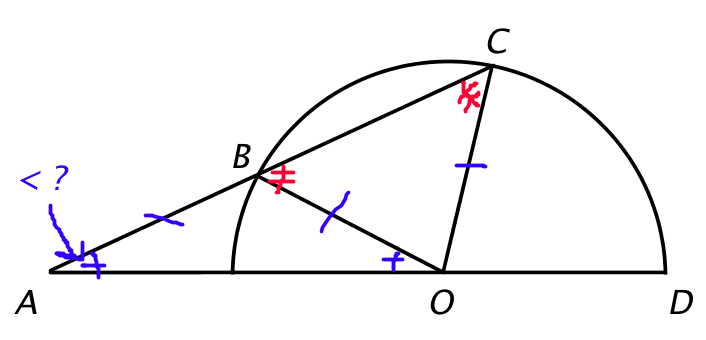Note, though, that there are two separate triangles here. We cant say that all four angles are the same measure, just two and two. I showed that above through different colors (red and blue). On the real test, of course, we dont get multi-colored pens, so youll have to use the classic geometry designation: two little slash marks instead of one to indicate a different angle.

Okay, anything else? I am noticing that we also have almost all of the angles for the big triangle ACO (two little blue ones at CAO and AOB, one little red one at BCO, and then angle COB). Im not sure whether thatll be useful; Im just noticing it for now.

Anything else? One more thing: this is DS, so its wise to copy the diagram over again at this point. Use one diagram for statement (1) and the second diagram for statement (2). That way, you wont mistakenly re-use information when youre not supposed to. Just remember: first, draw once and apply everything from the question stem (because you really can use that for both statements). Then, just before you try the statements, re-copy the full diagram.

Lets look at the first statement.

(1) The degree measure of angle COD is 60.

Add that in. Hmm. There are way too many angles going on here. Lets assign some variables.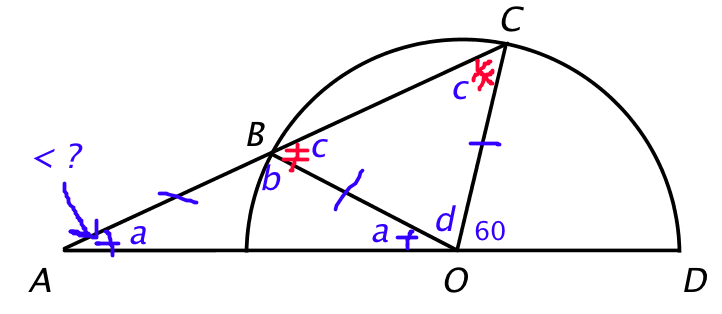Were trying to find a. What can we infer?

First, a + d + 60 = 180, so a + d = 120. Cool, thats one formula. Can we get any others?

Look at the big triangle, ACO. a + c + a + d = 180, or 2a + c + d = 180. We have a second equation, which is good, but we also added a third variable, which is not so good (not if we want to solve, anyway).

Is there a third equation? Oh, and is there a way to get a third equation using only the variables used so far (a, c, and d)? In other words, is there a third equation without using b?

There is! Do you remember the exterior angle rule for triangles? (If that rings a bell, try to articulate it before you keep reading.)

This rule basically says that, if you extend any side of a triangle and create an exterior angle (see below), then that exterior angle is equal to the sums of the two interior angles that dont touch the exterior angle. (Im going to call these opposite interior angles.)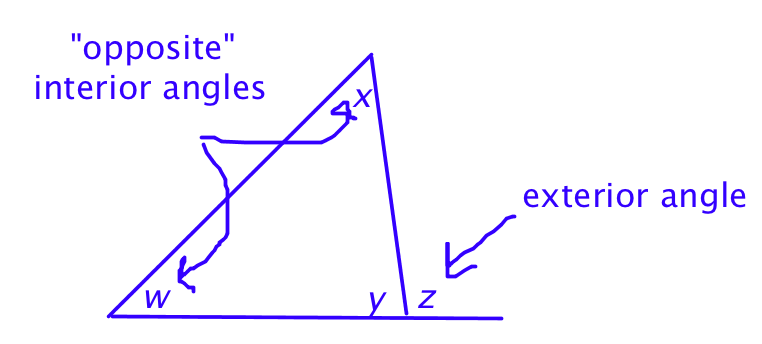Mathematically, the rule says that w + x = z.

Apply that to the problem: a + c = 60.

Excellent! We have three variables and three different, linear equations:

a + d = 120

2a + c + d = 180

a + c = 60

Its possible to solve for angle a! Statement (1) is sufficient.

This first statement is pretty nasty. Its crucial to infer from the information given in the question stem. The connection between the sidesand, therefore, the connection between certain anglesishow you might realize that this statement really is sufficient.

From there, youve also got to be able to see different relationships and write equations even though youve got all these weird shapes on top of each other. It might help, at that stage, to re-draw just the part of the diagram that you need. Just the big triangle would help to see these connections more clearly, like this: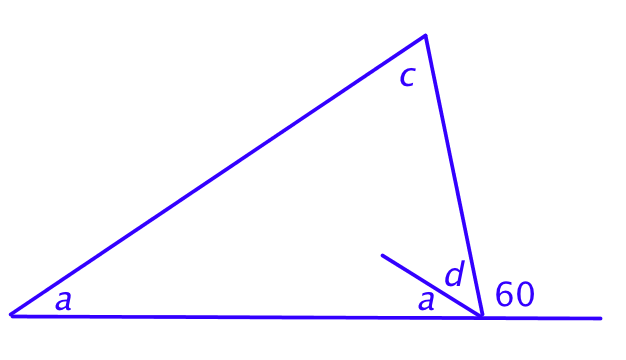All right, lets try statement (2).

(2) The degree measure of angle BCO is 40.

Remember, work from your clean second diagramnot the one that contains all the info from statement (1)!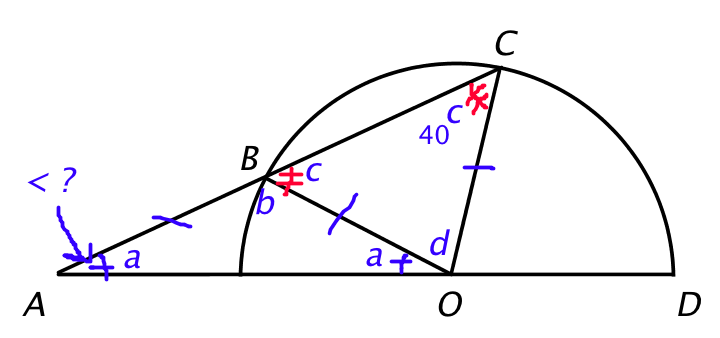So c = 40. Nice. But is that useful enough to get to a?

Hmm. If c = 40, we can find d, since c + c + d = 180.

Oh, hey! And look at the big triangle again. The big triangle is a + a + d + c = 180. If we know c and d, then we can find a. Done! Statement (2) is also sufficient.

That's crazy! Even though anyone would describe this shape as two triangles and a semi-circle, the one thing that really mattered was the big triangle made up of the two smaller ones. It was basically hidden in plain sight.

So there are two key takeaways related to multi-shape problems. First, as I mentioned towards the beginning, keep an eye out for any connections between the shapes; thats going to be one key to solving the problem. Second, pay attention not just to the individual or small shapes but to any larger shapes that they create. Its pretty common for side-by-side triangle problems to hinge on seeing the big third triangle created by the two smaller triangles.

I think theres a larger strategic takeaway on this one, too. It wasnt immediately obvious that statement (1) was going to be a lot harder (and require a lot more work) than statement (2), so I started with statement (1) when I did this for the first time myself. Then, I got hung up, so I went to (2) instead. By the time I finished doing statement (2), I was seeing the whole shape properly and I was able to make use of my new understanding to go back to statement (1) and figure it out.

## Key Takeaways for Knowing the Code:

(1) Be flexible! If something isnt working but you have something else you could do, jump down that other path instead. Even if switching to statement (2) hadnt later helped me on statement (1), Id have at least narrowed down my answers after statement (2).

(2) Be flexible within reason. You still have to hit a 2-minute-per-problem average on quant. Sometimes, you do have to let go and guess.

(3) Turn any knowledge you gain into Know the Code flash cards: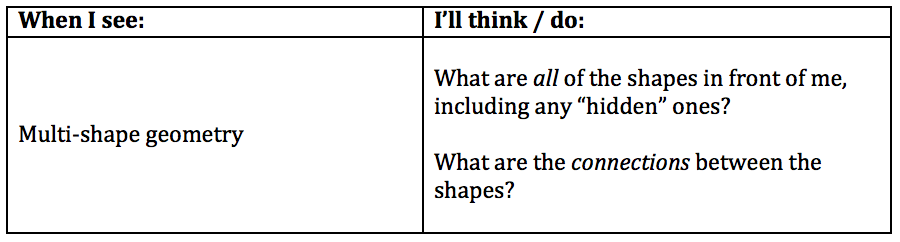* GMATPrep questions courtesy of the Graduate Management Admissions Council. Usage of this question does not imply endorsement by GMAC.